# ggalignment

## Introduction

The ggalignment package does one thing – it plot D&D-style alignment charts. If you’re unfamiliar, this is what they look like:

library(ggalignment)
library(dplyr)

ggalignment(alignment = data.frame(img = character(),
alignment = character()),
font_size = 3)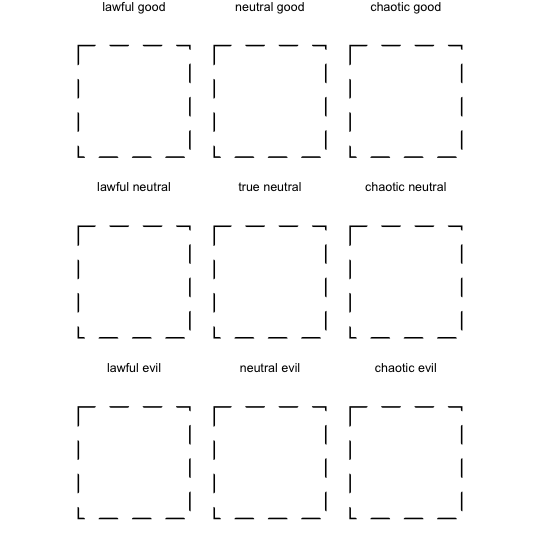Typically, each box contains an image of a person or thing. Each element of the chart should have a value in the x direction that represents how lawful or chaotic they are, and a value in the y direction that represents how good or evil they are. In this example, I’ve used some images of my cats and my perception of their alignments.

## Simple Plotting Alignment

To plot one image per box, you only need to pass in a data.frame containing two columns, img and alignment, which represent respectively the image path and the alignment name. For example:

align_cats <- example_cats()

If you pass this to the ggalignment() function, it will plot each of these images in the center of each box.

ggalignment(align_cats, font_size = 3)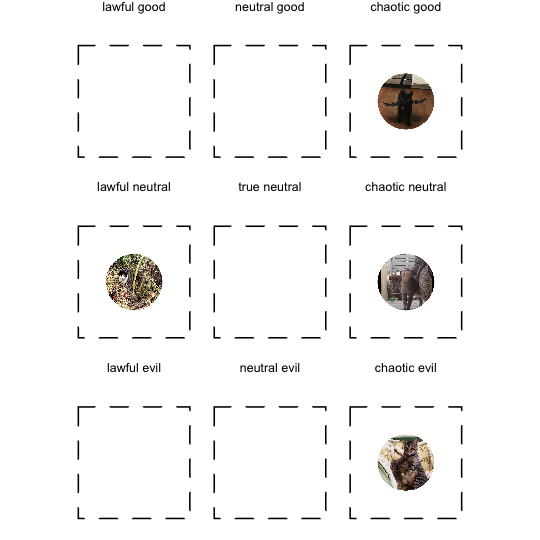You can also provide images of different shapes (not just circles) and these will likewise center on the origin or use the x and y columns if provided.

cats_rect <-
align_cats %>%
mutate(img = c(align_cats\$img[1:3],
system.file("img/gray_2.png", package = "ggalignment")))

ggalignment(alignment = cats_rect, font_size = 3)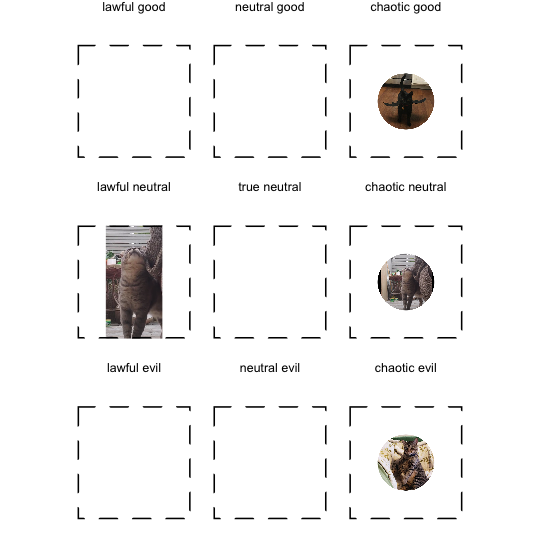## Plotting Alignment with Coordinates

If you have more than one image per alignment box, or if you would just like further control over the placement of the image in the box, you can also provide columns x and y in your alignment data passed to ggalignment() which will specify the x and y coordinates for each image.

Within each box, the coordinate system extends from -1 to 1 in both axes, and is centered at the origin. We can modify the default plot by providing x and y columns.

cats_with_coords <-
align_cats %>%
mutate(x = c(0.5, -0.5, -0.5, 0.5),
y = c(-0.5, -0.5, 0.5, 0.5))

ggalignment(alignment = cats_with_coords, font_size = 3)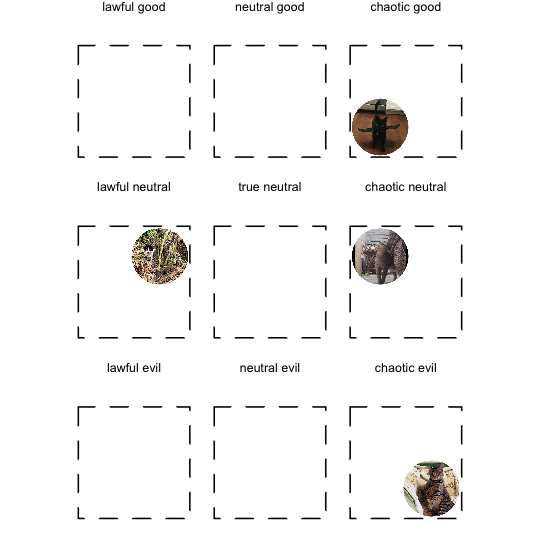This also works if there are multiple images per box, and x and y columns are required in this case to prevent two images occupying the same space at the origin and masking each other.

cats_with_multi_image <-
align_cats %>%
mutate(alignment = rep("chaotic neutral")) %>%
mutate(x = c(0.5, -0.5, -0.5, 0.5),
y = c(-0.5, -0.5, 0.5, 0.5))

ggalignment(alignment = cats_with_multi_image,
font_size = 3)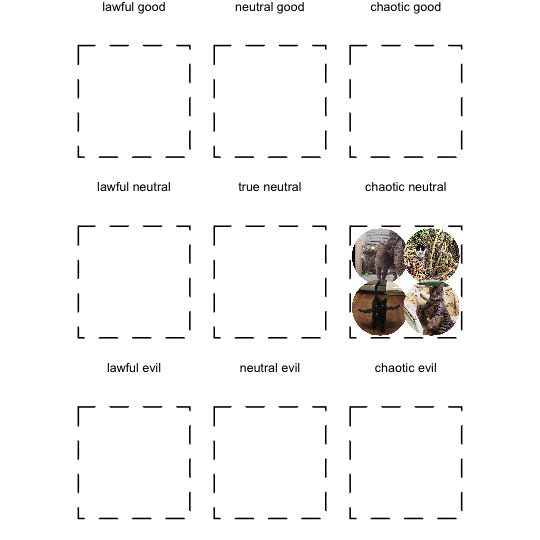## Controlling Image Size

You can adjust the image size using the parameters max_images_per_dim (defaults to “width”) and max_image_dim. max_images_per_dim controls how many images will fit per facet, defaulting to how many fit width-wise. Depending on the ratio of your images, you may want to switch max_images_dim to “height”, which will control how many images will fit per facet height-wise. Generally, you should pick the dimension that is larger.

These two parameters allow you to adjust the image size. For example, if I use max_images_per_dim = 1 with the default setting of max_image_dim = "width", I will get an image that is the full width of the facet box.

ggalignment(alignment = align_cats,
font_size = 3,
max_images_per_dim = 1)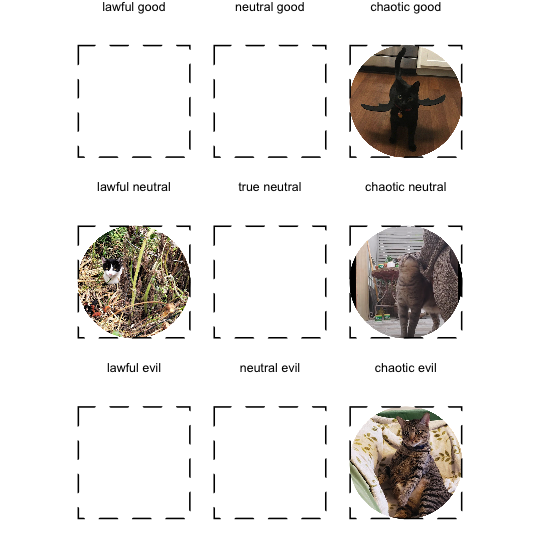Setting max_images_per_dim = 2 will cause the images to take up 1/2 the width of the facet box.

ggalignment(alignment = align_cats,
font_size = 3,
max_images_per_dim = 2)Likewise, max_images_per_dim = 4 will yield images that are 1/4 the width of the facet box. Controlling the image size is useful if you have a lot of images in a single box, and the default of max_images_per_dim = 2 will lead to overcrowding.

ggalignment(alignment = align_cats,
font_size = 3,
max_images_per_dim = 4)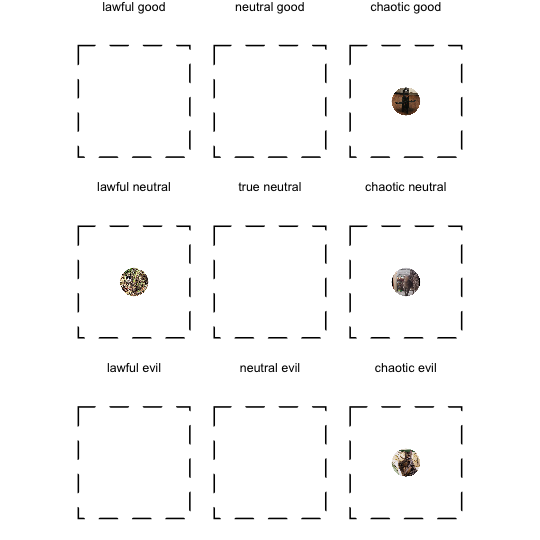## Customizing the Look

You can change many things about the look of the chart, including for the full list of acceptable arguments. font size, font family, font color, background color, line color, and line type for the facets. These are all passed to ggplot(), so see the documentation there

ggalignment(alignment = align_cats,
line_type = "dotted",
line_color = "seagreen1",
background_color = "turquoise4",
font_color = "skyblue1",
font_size = 3)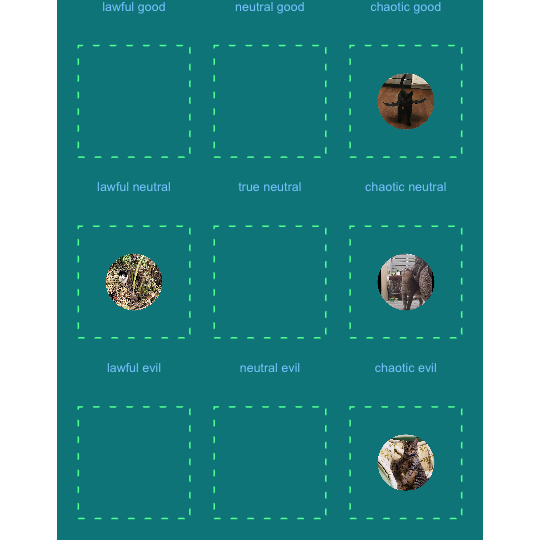If you want to use your own image files, just construct a data.frame containing an img column containing the file paths, an alignment column containing the alignments, and optionally x and y columns determining the location of each image within its alignment facet. If you have multiple images in a single alignment, x and y are required.

First, let’s look at an example with local files. We’ll use the R logo and a portrait of my cat Oberon (thank you to artist ChargedAsylum for the portrait!).

local_example <-
data.frame(img = c("r_logo.png",
"obie_portrait.png"),
alignment = c("lawful good",
"chaotic neutral"))

ggalignment(local_example, font_size = 3)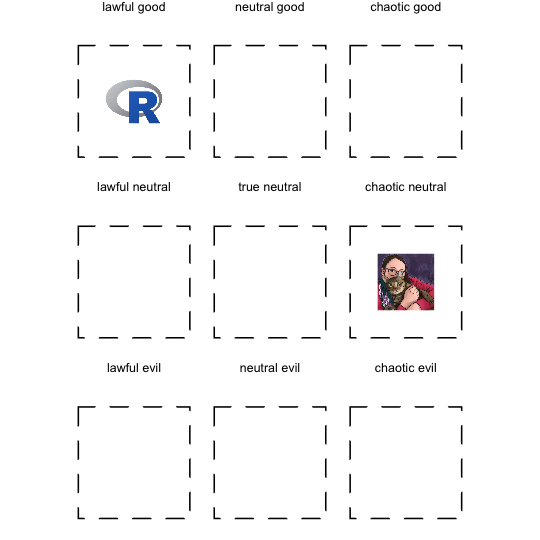You can also use a URL as your image path.

url_example <-
data.frame(img = c("https://www.r-project.org/Rlogo.png",
"https://avatars.githubusercontent.com/u/18043377?v=4"),
alignment = c("lawful good",
"chaotic neutral"))

try({ # wrapped with a try block here to prevent CRAN issues
ggalignment(url_example, font_size = 3)
})